# Triangles: Finding Their Areas

In this worksheet, students calculate the area of a triangle by considering it as half of a rectangle.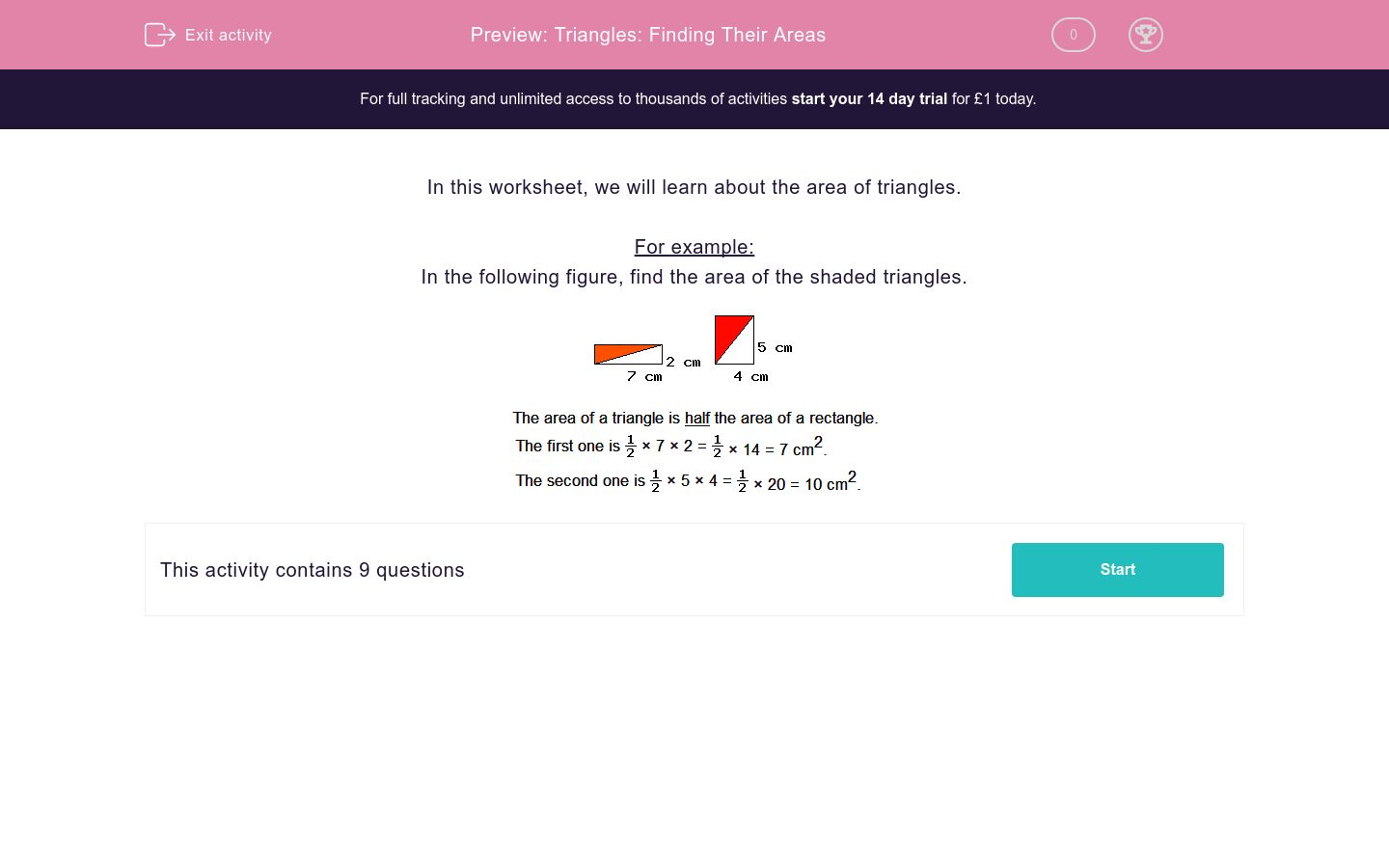Key stage:  KS 2

Curriculum topic:   Maths and Numerical Reasoning

Curriculum subtopic:   2D Shapes: Triangles, Quadrilaterals and Polygons

Difficulty level:### QUESTION 1 of 10

In this worksheet, we will learn about the area of triangles.

For example:

In the following figure, find the area of the shaded triangles.Find the shaded area of this triangle.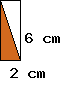The shaded area is __________ cm2

Find the shaded area of this triangle.The shaded area is __________ cm2

Find the shaded area of this triangle.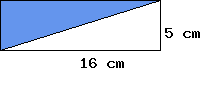The shaded area is __________ cm2

Find the shaded area of this triangle.The shaded area is __________ cm2

Find the shaded area of this triangle.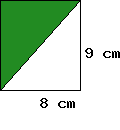The shaded area is __________ cm2

Find the shaded area of this triangle.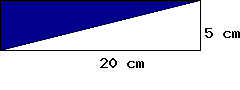The shaded area is __________ cm2

Find the shaded area of this triangle.The shaded area is __________ cm2

Find the shaded area of this triangle.The shaded area is __________ cm2

Find the shaded area of this triangle.The shaded area is __________ cm2

• Question 1

Find the shaded area of this triangle.The shaded area is __________ cm2

6
EDDIE SAYS
6 x 2 = 12

12 ÷ 2 = 6

• Question 2

Find the shaded area of this triangle.The shaded area is __________ cm2

70
EDDIE SAYS
20 x 7 = 140

140 ÷ 2 = 70

• Question 3

Find the shaded area of this triangle.The shaded area is __________ cm2

40
EDDIE SAYS
16 x 5 = 80

80 ÷ 2 = 40

• Question 4

Find the shaded area of this triangle.The shaded area is __________ cm2

135
EDDIE SAYS
18 x 15 = 270

270 ÷ 2 = 135

• Question 5

Find the shaded area of this triangle.The shaded area is __________ cm2

36
EDDIE SAYS
9 x 8 = 72

72 ÷ 2 = 36

• Question 6

Find the shaded area of this triangle.The shaded area is __________ cm2

50
EDDIE SAYS
20 x 5 = 100

100 ÷ 2 = 50

• Question 7

Find the shaded area of this triangle.The shaded area is __________ cm2

15
EDDIE SAYS
10 x 3 = 30

30 ÷ 2 = 15
• Question 8

Find the shaded area of this triangle.The shaded area is __________ cm2

32.5
EDDIE SAYS
13 x 5 = 65

65 ÷ 2 = 32.5

• Question 9

Find the shaded area of this triangle.The shaded area is __________ cm2

110.5
EDDIE SAYS
17 x 13 = 221

221 ÷ 2 = 110.5

---- OR ----

Sign up for a £1 trial so you can track and measure your child's progress on this activity.

### What is EdPlace?

We're your National Curriculum aligned online education content provider helping each child succeed in English, maths and science from year 1 to GCSE. With an EdPlace account you’ll be able to track and measure progress, helping each child achieve their best. We build confidence and attainment by personalising each child’s learning at a level that suits them.

Get started